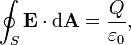Home » Posts tagged 'magnitude'

# Tag Archives: magnitude

## What is the answer if vector A is multiplied with vector B?

In vector multiplication, there are two types of multiplication: One in which the result is a scalar and the other in which the result is a vector.

Accordingly the two types of products of vectors are:

Scalar product (also called dot product) denoted byA.B

Vector product (also called cross product) denoted by AxB

A.B  = AB cos Θ, where Θ is the angle between the directions of A and B

The physical meaning of dot product is that, “The product of the magnitude of one vector and the magnitude of the component of the other vector in the direction of the first vector.”

AxB = AB sinΘ$\hat{n}$

The direction of cross product is given by right hand cork screw rule## Numerical from Kinematics and vectors

1. A jetliner moving at 500. km/h due east moves into a region where the wind is blowing from exactly NW toward the SE at a speed of 127 km/h. What is the new velocity of the airplane? you must include both magnitude and direction in your answer.
2. The speed of a train changes from36km/h to 72km/h in 10sec. calculate the distance travelled during this time

## Doubts related to Electric Dipole

1. Magnitude and direction of dipole field depends not only on distance r from centre of dipole,but also on the angle between the position vector rand the dipole moment p how?
2. How can centres of positive and negative charge lie on same place and if both have same charge they should become neutral?

1. The electric field at a point due to a dipole is given by$\hat{n}$

2. Centre of positive charge means the centre of mass of all the positive charged particles and similarly for the negative charge. If the atom or molecule is symmetric, the two centres of charge will coincide; just like the centre of mass of two concentric hollow spheres. The charges are not at the same point, but their centres of mass coincide. If they do not coincide, it will give rise to an electric dipole or a polar molecule.

## Why Electric Current is a Scalar Quantity?

“Why is current a scalar quantity even after having both magnitude as well as direction?”

For a physical quantity to be termed a vector quantity, having magnitude and direction is not enough. The quantity should obey the laws of vector addition too. Like the triangle law or the parallelogram law. As we know, if two currents meet at a junction, the total current of the resultant current will be the algebraic sum of the two current and not the vector sum.

1. the drawing on the left shows two vectors A and B, and the drawing on the right shows their components.The scalar components of these vectors are:When the vectors A and B are added, the resultant vector is R, so that RAB. What are the x– and y-components of R?
2. the displacement vectors A and B, when added together, give the resultant vector R, so that RAB. Use the data in the drawing to find the magnitude R of the resultant vector and the angle q that it makes with the +x axis.In the first question as you can see that the X components (Ax and Bx being equal and opposite will cancel each other and therefore the X com ponent of the resultant is zero.

The Y components will add up and therefore the Y component of resultant = 3.4+3.4 = 6.8 m and this itself is the magnitude of the resultant as the X components have canceled out.

Hnit for Answer to the second question## Gauss Theorem – Electrostatics

“WHAT IS THE  MAGNITUDE OF ELECTRIC  FIELD IN THE  GAUSSIAN SURFACE OF  A CUBE,AT ITS FACE ,AT ITS CENTRE, AT ITS CORNERS OR AT ANY OTHER POINT INSIDE THE CUBE. ALSO TELL ME THE THE WAY TO KNOW IT.”

Ans:

According to Gauss Theorem, the total electric flux through a closed surface,The Gaussian surface is an imaginary surface. So, for calculating the electric field at a point using Gauss theorem, we have to imagine a Gaussian surface symmetric with the given charge distribution.

I have assumed that there is a point charge Q at the centre of the cube.

At its face (at a point on the face on the line radially outwards from the point charge at the centre of the cube and perpendicular to the face)

The distance is equal to half the length of side of the cube (Say L).

On the corner,

Calculate the distance from the centre of the cube to its corner and replace (L/2) in the above equation with that distance.

### Hits so far @ AskPhysics

• 2,182,805 hits

### CBSE Exam Count Down

AISSCE / AISSE 2020February 26, 2020
37 days to go.